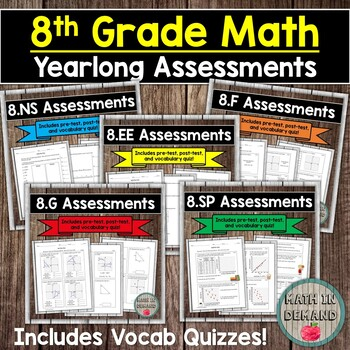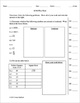# 8th Grade Math Assessments EDITABLE (Entire Year) DISTANCE LEARNINGSubject
Resource Type
File Type

Zip

(3 MB|40 (80 including answer keys))
Also included in:
1. This bundle includes ALL of my math curriculum in my Teachers Pay Teachers store. You will be receiving all of my 6th, 7th, and 8th grade activities, exit slips, assessments, interactive notebooks, foldables, and much more! This is well over 1000 pages of material that I have created to help make
\$1365.72
\$559.20
Save \$806.52
2. This is my 8th grade math bundle. Everything included in this bundle is my 8th grade resources in my store. This includes my 8th grade math interactive notebook, warmups, activities, exit slips, foldables, practice worksheets, vocabulary coloring worksheets, homework, and assessments (pre-tests, p
\$359.42
\$159.99
Save \$199.43
Product Description

This is a bundle including all of my 8th grade math assessments. This includes a pre-test, post-test, and vocabulary quiz for every standard: 8.NS, 8.EE, 8.F, 8.G, and 8.SP. They are now EDITABLE!!! Hence, you can decide if you want to add more rigor for your advanced classes or keep it more simple! It is up to you if you want to change any of the problems!

Included is a pre-test, post-test, and vocabulary quiz on 8.NS (8th grade Common Core standard - Number System).

Students will:

1.) Determine if numbers are rational or irrational.

2.) Fill out a table containing perfect squares.

3.) Approximate square roots that are irrational numbers.

4.) Use a number line to show where irrational numbers lie on the line.

5.) Determine the closest number to the square root of an irrational number.

5.) Convert fractions to decimals.

6.) Convert repeating decimals to fractions.

The vocabulary included is square root, whole number, repeating decimal, real number, irrational number, rational number, perfect square, natural number, and terminating decimal.

Included is a pre-test, post-test, and 2 vocabulary quizzes on the 8th grade Common Core Standards Expressions & Equations (8.EE).

Students will:

1.) Use the law of exponents to simplify expressions

2.) Solve square roots

3.) Convert numbers to scientific notation

4.) Convert numbers to standard form

5.) Determine the slope, y-intercept, and slope-intercept form of a line

6.) Solve a system of equations

7.) Graphing a system of equations

The vocabulary included is base, exponent, expression, monomial, coefficient, scientific notation, standard form, slope-intercept form, variable, linear, distributive property, system of equations, point of intersection, elimination, substitution, unit rate, slope, and y-intercept.

Included is a pre-test, post-test, and vocabulary quiz on the 8th grade math standard functions (8.F).

Students will:

1.) Determine if a graph represents a function

2.) State the domain and range of a relation

3.) Plot points on a graph to determine if the table represents a function

4.) State if a function is decreasing, increasing, or constant

5.) Determine the output of a function machine

6.) Determine the recursive and explicit equation

7.) Determine the minimum, maximum, increasing interval, and decreasing interval of a graph

8.) Determine the rate of change, initial value, independent value, and dependent variable given a graph

9.) Sketch a graph given a situation

The vocabulary included is dependent, output, function, domain, range, decreasing function, input, range, non-linear function, relation, increasing function, and function notation.

Included is a pre-test, post-test, and vocabulary quiz on the Common Core 8th grade standard Geometry (8.G).

Students will:

1.) Perform transformations on figures such as reflections, rotations, dilations, and translations.

2.) Determine if two figures are similar.

3.) Determine if two figures are congruent.

4.) Determine the missing angle inside of a triangle.

5.) Determine the remote exterior angle.

6.) Use the Pythagorean Theorem to determine a missing length in a right triangle.

7.) Calculate the volume of three dimensional objects: cone, cylinder, and sphere.

The vocabulary included is rotation, reflection, dilation, translation, remote interior angle, exterior angle, Pythagorean Theorem, corresponding angles, similar figures, transversal, and congruent.

Included is a pre-test, post-test, and vocabulary quiz on 8.SP (8th grade Common Core standard - Statistics & Probability).

Students will:

1.) Construct a scatter plot.

2.) Describe the associations of scatter plots.

3.) Determine if given lines are an accurate line of fit for scatter plots.

4.) Draw a line of best fit.

5.) Write an equation in slope-intercept form for the line of best fit.

6.) Determine the association given word problems.

7.) Use a two-way table to determine the percent of preference.

The vocabulary included is line of best fit, cluster, nonlinear association, linear association, negative association, positive association, outlier, scatter plot, and bivariate measurement data.

Total Pages
Included
Teaching Duration
1 Year
Report this Resource to TpT
Reported resources will be reviewed by our team. Report this resource to let us know if this resource violates TpT’s content guidelines.\$23.99
List Price:
\$29.99
You Save:
\$6.00